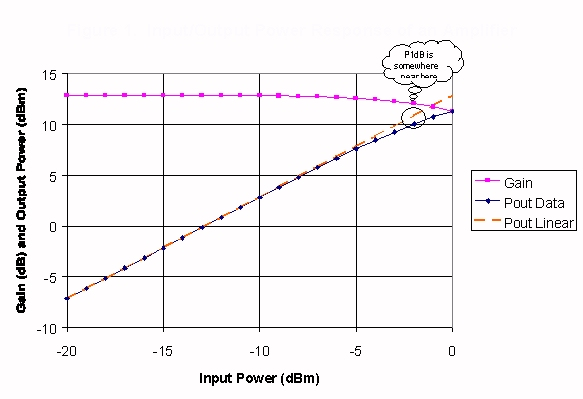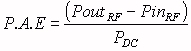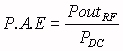# Non-Linear Devices

Non-linear means that the relationship between input power (or voltage) and output power does not plot on a graph as a straight line. Don't confuse non-linearity with frequency response (gain versus frequency). In general, passive components are purely linear (loss is constant versus input power), while active components are all nonlinear.

Also, it is OK to spell "nonlinear" as "non-linear", in our opinion. This site suggests that adding the hyphen is British English, while omitting it is American English. Here at Microwaves101 we swing both ways!

Here's a page we've started on nonlinear transmission lines  --and we've added a link to a video of a soliton!

Here's a page on nonlinear passive networks

Know the difference between small signal response and large signal response. Small-signal implies that the RF signal is low enough so that the device behaves linearly, i.e. a one dB increase in input power results in a one dB increase in output power. Large-signal means that you have enough RF signal so that the output is straining to match the input dB for dB, and in the case of an amplifier, the DC quiescent point may actually be shifted due to drive level.

Amplifier nonlinearity

Mixer nonlinearity

Limiter nonlinearity

Multiplier nonlinearity

Gain compression points

### Amplifier nonlinearity

Amplifiers are nonlinear, active devices. At small-signal levels, the transfer characteristic is constant with drive level, and is equal to the small-signal gain of the device. At higher power levels, the amplifier's power gain is reduced, and is said to enter gain compression. At some power level the output power saturates, and no additional power results at the output as the input is driven even harder. The familiar input/output power characteristic of an amplifier is shown below:Because they are active devices, amplifiers require one or more power supplies. The efficiency of an an amplifier is measured in two ways. Power-added efficiency (PAE) is calculated as the output power minus the input power, divided by the DC power. Drain efficiency is calculated as the output power divided by the DC power ("drain" implies that we are discussing a FET amplifier). Note that the drain efficiency is always higher than the power-added efficiency. However, for amplifiers with high gain (30 dB for example), the PAE is nearly equal to the drain efficiency. Efficiency is a function of many things, including operating voltage, class of operation (A, B, AB, etc), drive level, frequency, temperature, etc.Hey this should say "drain efficiciency!### Mixer nonlinearity

A mixer is used to translate one frequency to another. It is a passive device, meaning that no DC power is applied. It is also a nonlinear device, meaning that for small signals it will exhibit constant conversion loss (much like an amplifier exhibits constant gain) , but at large signals the response will compress and then saturate. In this respect it is similar to an amplifier.

### Limiter nonlinearity

Moved to a separate page.

### Multiplier nonlinearity

A frequency multiplier has a much different power transfer characteristic compared to an amplifier, mixer or limiter. Usually a multiplier follows what is called a "square-law" curve, meaning that the output power is proportional to the input power (in watts) squared.